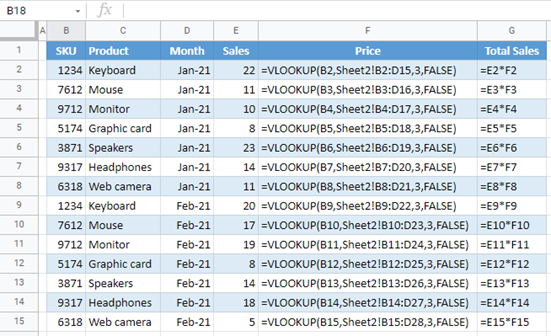# How to Put Excel & Google Sheets in Formula View Mode

This tutorial demonstrates how to put Excel and Google Sheets in formula view mode.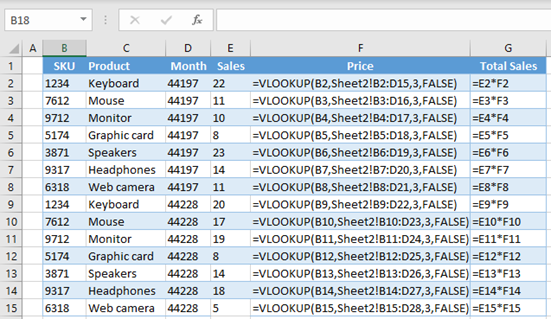## Put Excel in Formula View Mode

In Excel, you can view a formula by selecting the cell it’s in. However, in some cases, it can be useful for you to see all the formulas in the worksheet. For example, if you want to change something in a formula when the formula view is enabled, you can use Find & Replace to change all formulas at once.

Say you have the following example with formulas in Columns F and G (Price and Total Sales). In Column F, there is a VLOOKUP formula, while in Column G, the result is a multiplication of values from Columns E and F.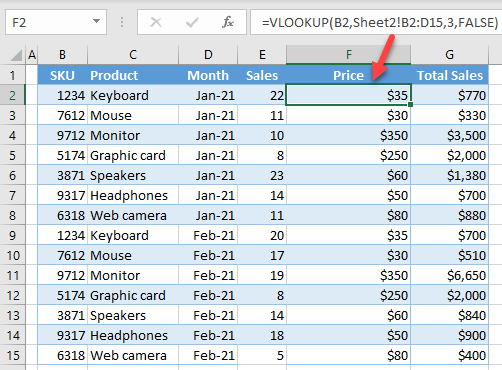▸ To enable formula view mode, in the Ribbon, go to Formulas > Show Formulas (or use the keyboard shortcut CTRL + `).

Note that the ` character is the grave accent, on the same key as the ~ (tilde).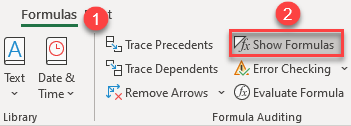Now, formulas are displayed as text, and dates are shown as serial numbers.Note: If you want to turn off the formula view and get back to the normal view, just follow the same path again and click Show Formulas.

## Put Google Sheets in Formula Mode View

You can also show formulas on the sheet.

▸ In the Menu, go to View > Show Formulas (or use CTRL + `).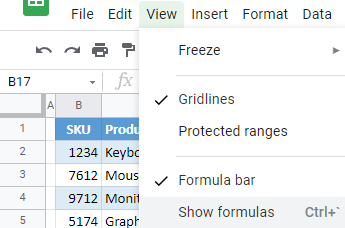As a result, all formulas in the sheet are displayed.